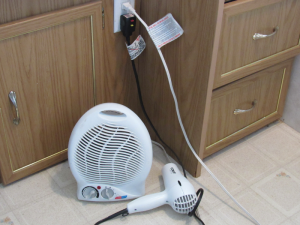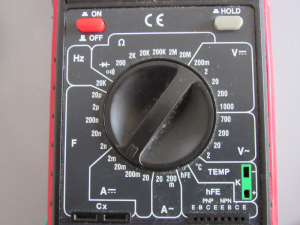# Calculating Power

## Introduction to Calculating Power (Watts)

If you are not familiar with calculating resistance or current, please visit these posts.   This will help you better understand basic electrical principals before trying to calculate power.

The Unit of power is the WATT.    Power itself is easy to calculate, but we will do a few extra calculations so you can see how power relates to other parameters such as resistance and current.

## Power Formula

The formula for power states that P=VI where P is power in watts, V is voltage (in volts), and I is current (in amps).   As long as we know the voltage and current, we can easily find power.   Remember, though, the circuit breaker in your house is rated in Amps (current).  We can use some other modifications of the power formula to find the amps or volts.   Let’s look at these variations which are manipulated algebraically from the main power formula.

P=VI    —   V=P/I  —  I=P/V

In the last circuit of our post on calculating current, we applied 5V across a certain resistance, and our result was .33mA.   Therefore, we would say that the power of our circuit is 5v x .00033, which is 0.00165 watts, or 1.65 MilliWatts.

Consider a circuit in our house though.   Here we have a hair dryer, and a heater:The Dryer consumes 1300 Watts, and the heater consumes 1500 Watts.  The circuit breaker for this circuit is 15A.   Will it trip the circuit breaker if both devices are energized at the same time?   Let’s do some math and figure it out.

The total power for this circuit is 2800 watts.   To find the current, we will use the formula:  I=P/V.   Household voltage here in the US is 120 Volts.   Therefore, Current = 2800/120.   We will draw 23.333 Amps.   The circuit breaker WILL trip.

The heater does have a low setting of 900 Watts.   If I turn the heater on low (900 watts), and the dryer on high (1300 watts), will the breaker trip?

In this case, we would only be using 2200 watts total, but 2200/120 is still 18 amps, so we are still drawing too much current.

Let’s try one more calculation on your own:  If I set the hair dryer on low (600 watts), and the heater on low (900 watts), will my circuit trip?

In this case, we are only pulling 1500 watts total.   1500/120 is only 12.5 Amps.   Using these formulas, you can calculate how many appliances you can place on a breaker without it tripping.You can purchase a good quality ammeter, but remember, when measuring amps, the meter will be connected in series with the circuit.   To minimize the effect on the circuit, the ammeter will have a very low resistance (acting as a short), so be sure it is connected properly, set for the proper range that you would expect, and that the meter is rated for the amperage that you are measuring.